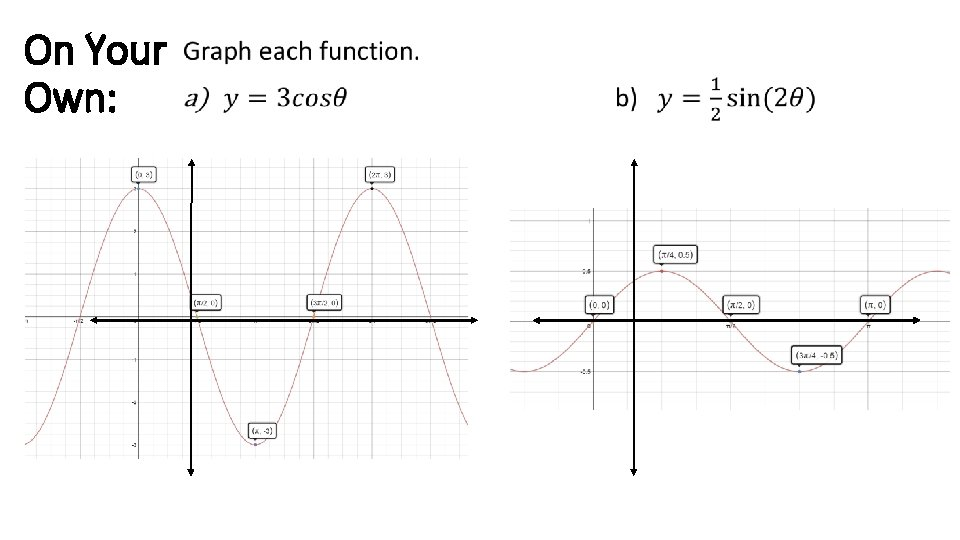# Trigonometric Functions Angles in Standard Position An angle

• Slides: 43Trigonometric FunctionsAngles in Standard Position • An angle on the coordinate plane is in standard position if the vertex is at the origin and one ray is on the positive x-axis • The ray on the x-axis is called the initial side of the angle • The ray that rotates about the center is called the terminal side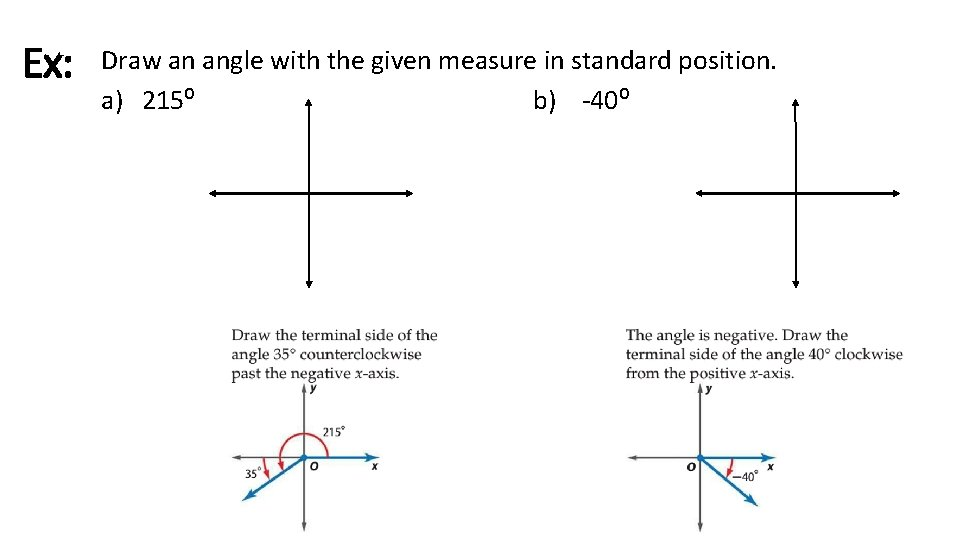Ex: Draw an angle with the given measure in standard position. a) 215⁰ b) -40⁰Ex: Wakeboarding is a combination of surfing, skateboarding, snowboarding, and water skiing. One maneuver involves a 540 -degree rotation in the air. Draw an angle is standard position that measures 540⁰.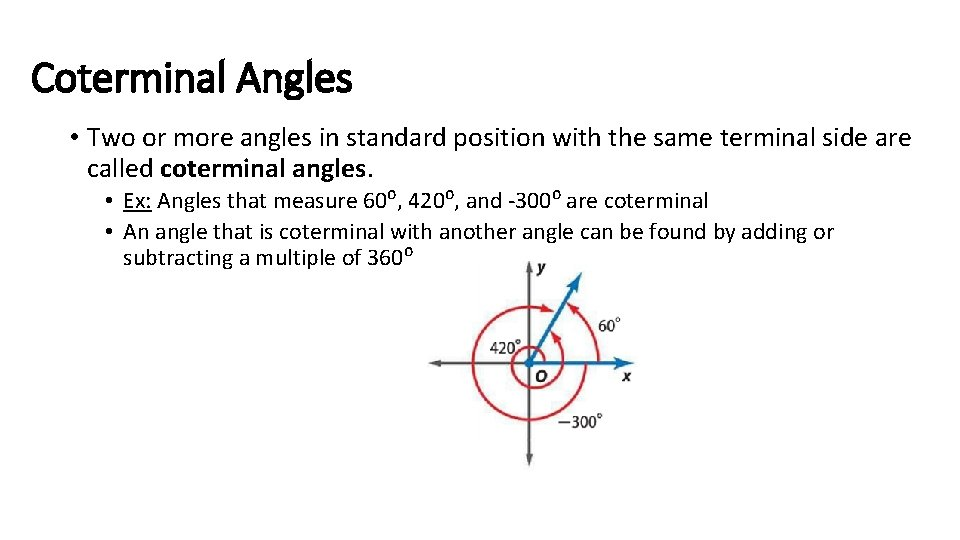Coterminal Angles • Two or more angles in standard position with the same terminal side are called coterminal angles. • Ex: Angles that measure 60⁰, 420⁰, and -300⁰ are coterminal • An angle that is coterminal with another angle can be found by adding or subtracting a multiple of 360⁰Ex: Find an angle with a positive measure and an angle with a negative measure that are coterminal with each angle. a) 130⁰ b) -200⁰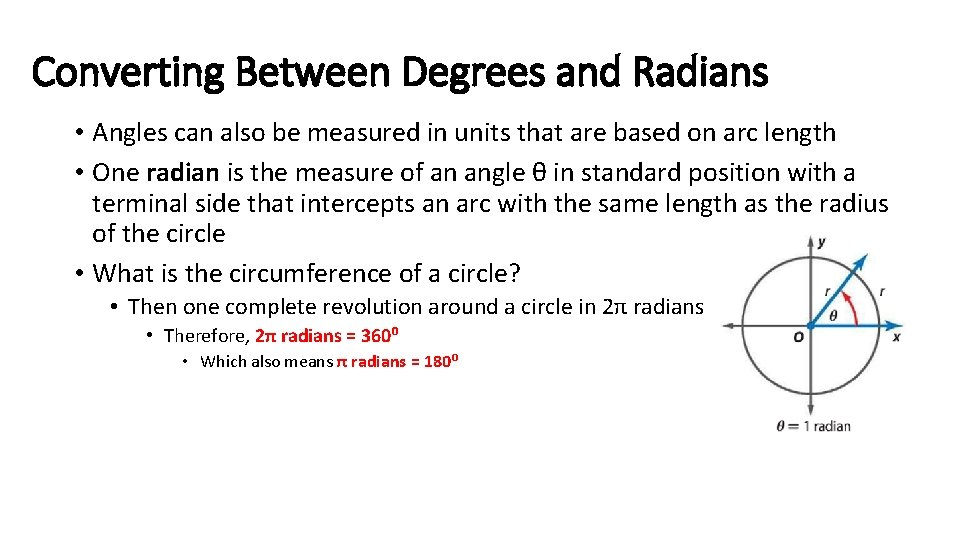Converting Between Degrees and Radians • Angles can also be measured in units that are based on arc length • One radian is the measure of an angle θ in standard position with a terminal side that intercepts an arc with the same length as the radius of the circle • What is the circumference of a circle? • Then one complete revolution around a circle in 2π radians • Therefore, 2π radians = 360⁰ • Which also means π radians = 180⁰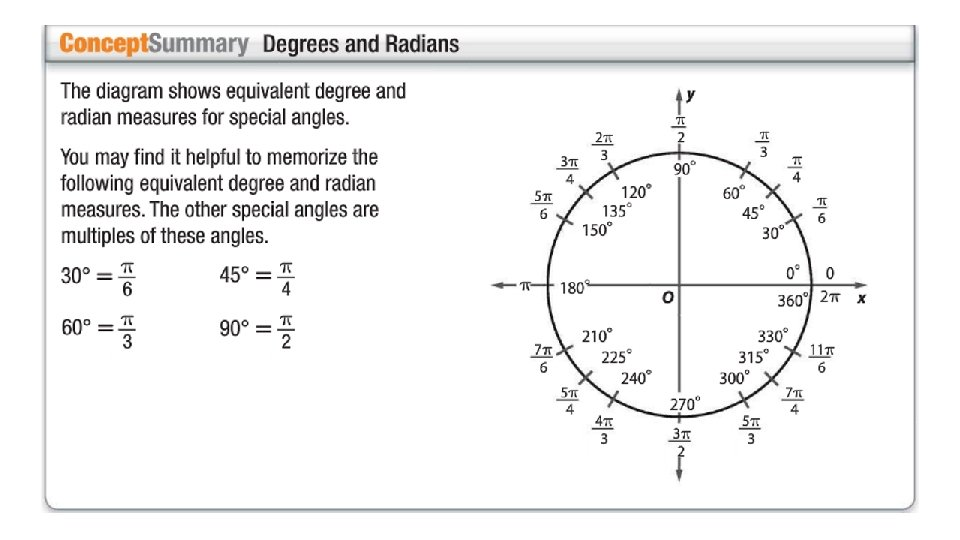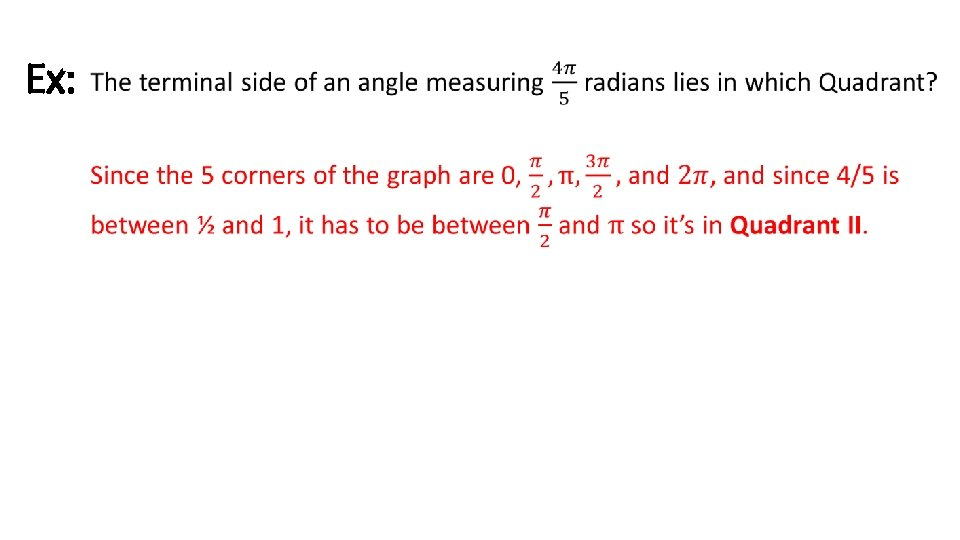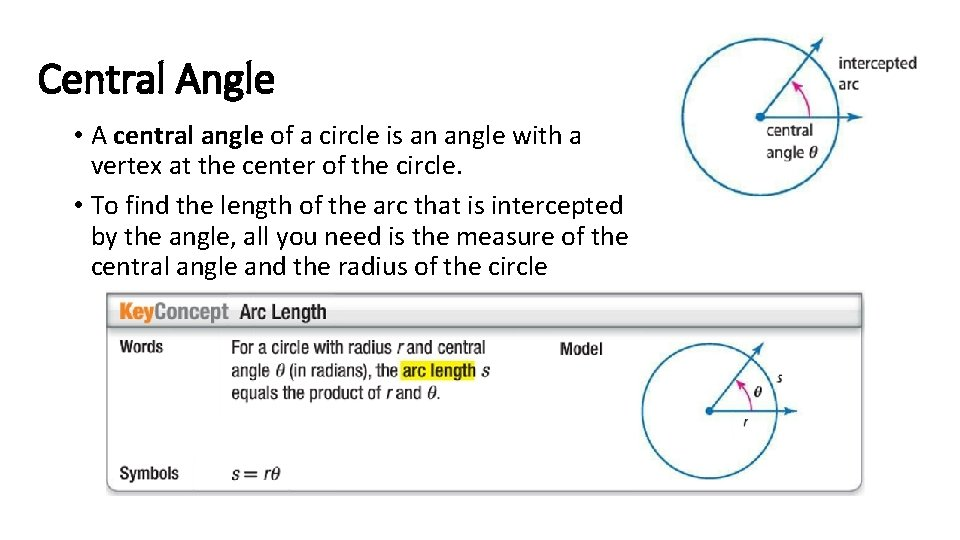Central Angle • A central angle of a circle is an angle with a vertex at the center of the circle. • To find the length of the arc that is intercepted by the angle, all you need is the measure of the central angle and the radius of the circle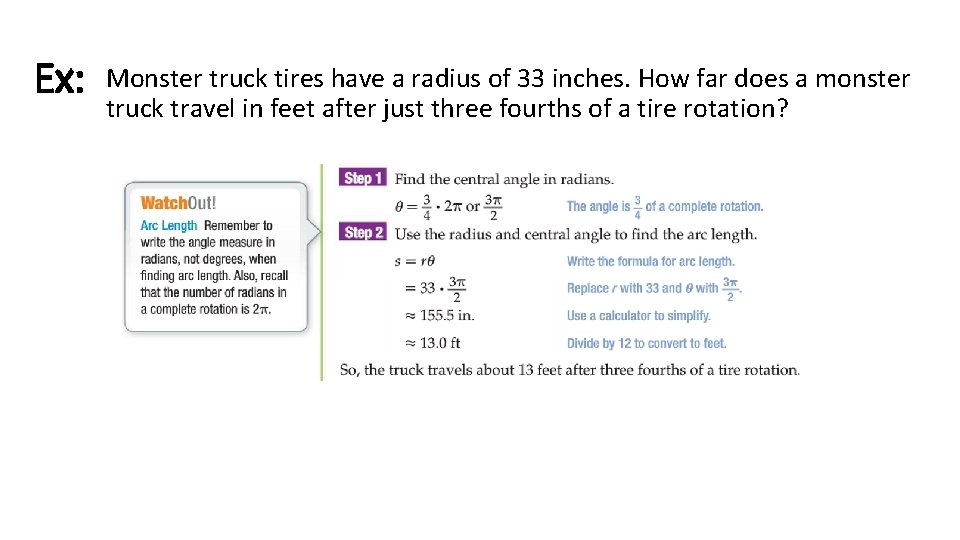Ex: Monster truck tires have a radius of 33 inches. How far does a monster truck travel in feet after just three fourths of a tire rotation?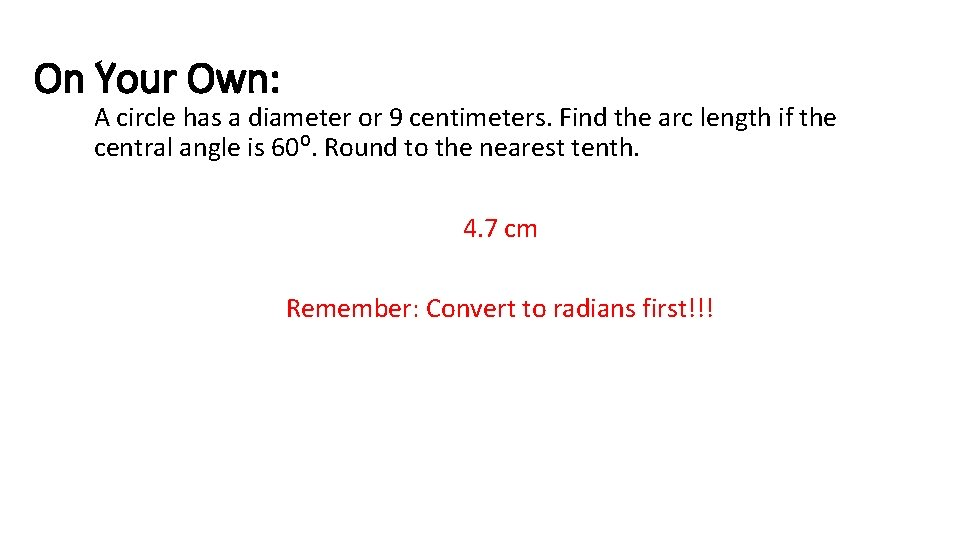On Your Own: A circle has a diameter or 9 centimeters. Find the arc length if the central angle is 60⁰. Round to the nearest tenth. 4. 7 cm Remember: Convert to radians first!!!SOHCAHTOA • The main 3 trigonometric functions!!!Unit Circle •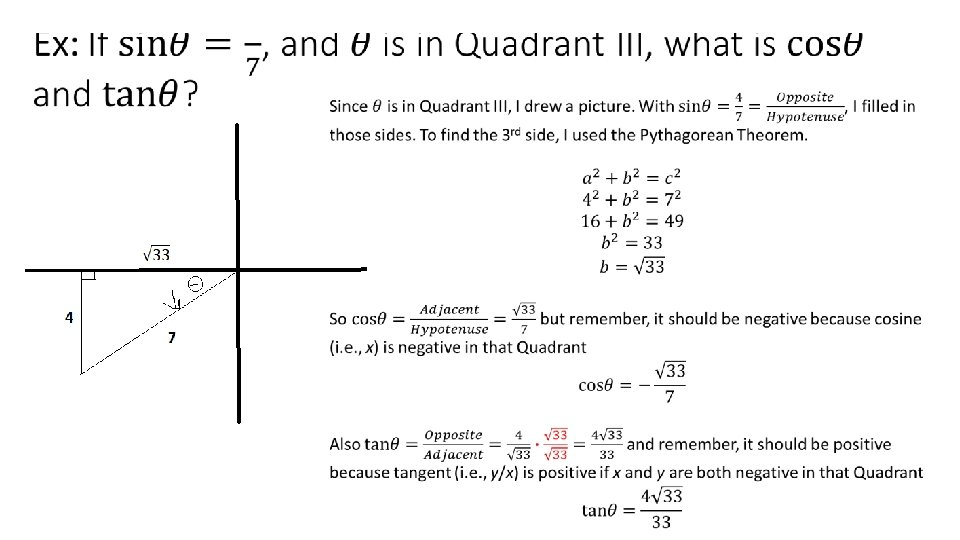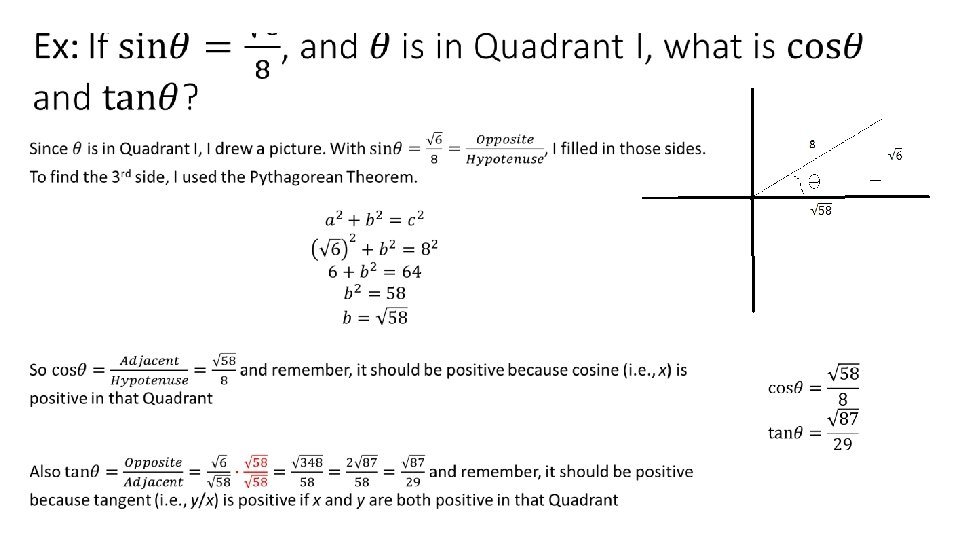Periodic Functions • A periodic function has y-values that repeat at regular intervals. One complete pattern is a cycle, and the horizontal length of one cycle is a period.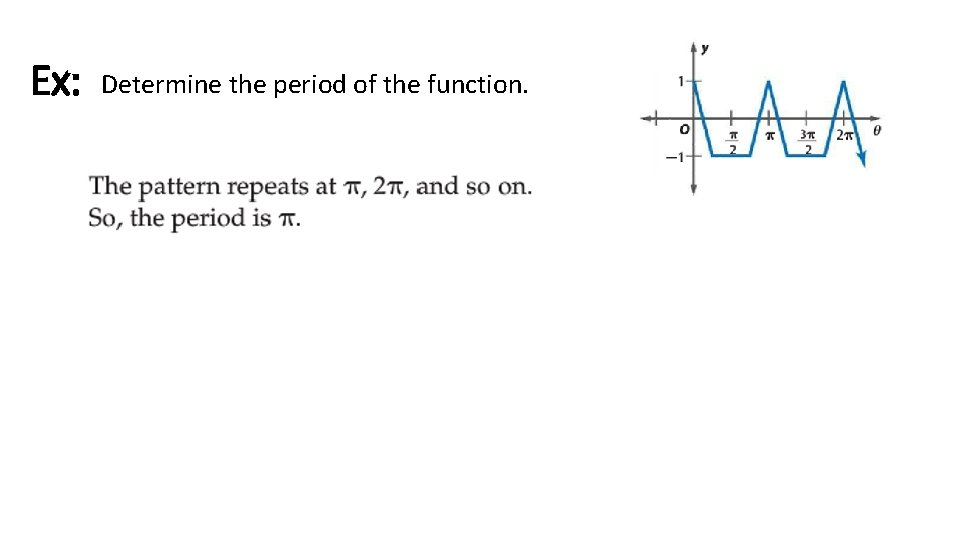Ex: Determine the period of the function.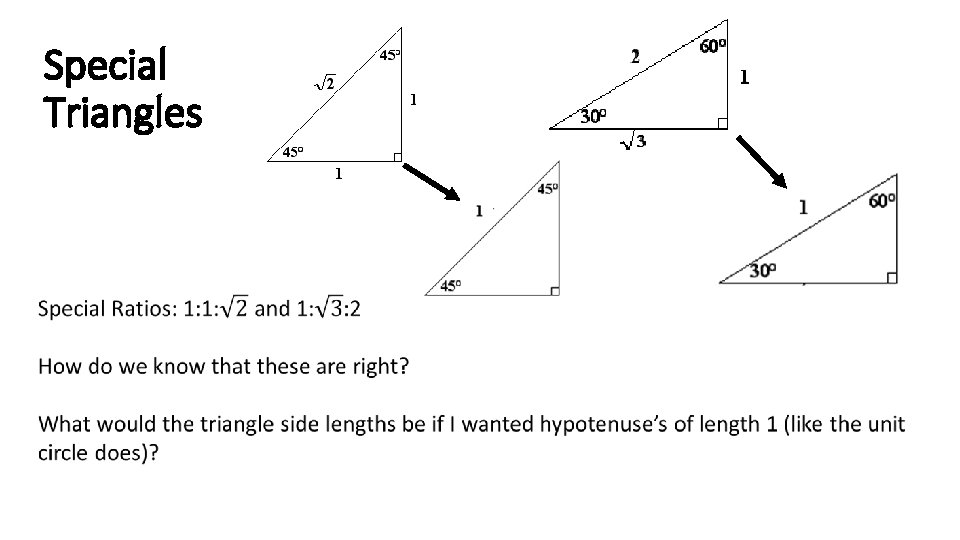Special Triangles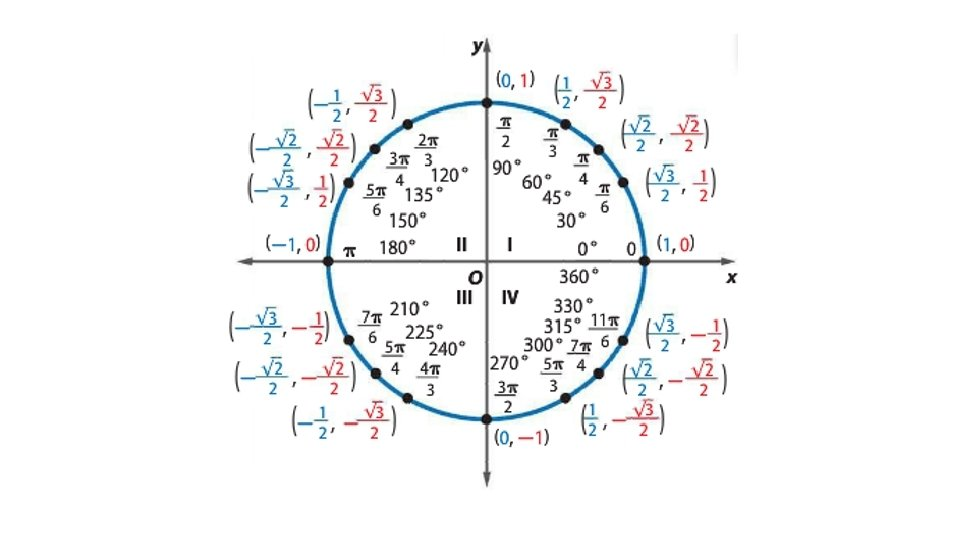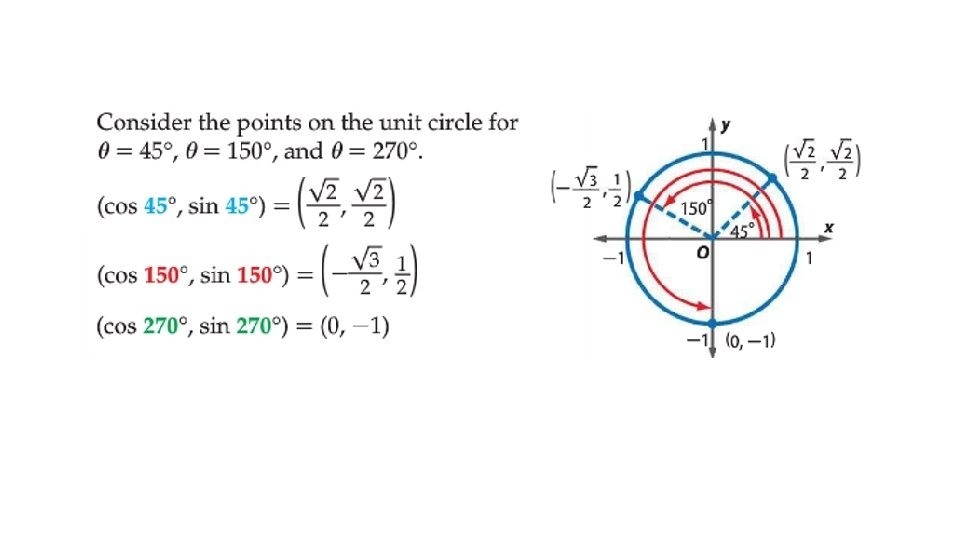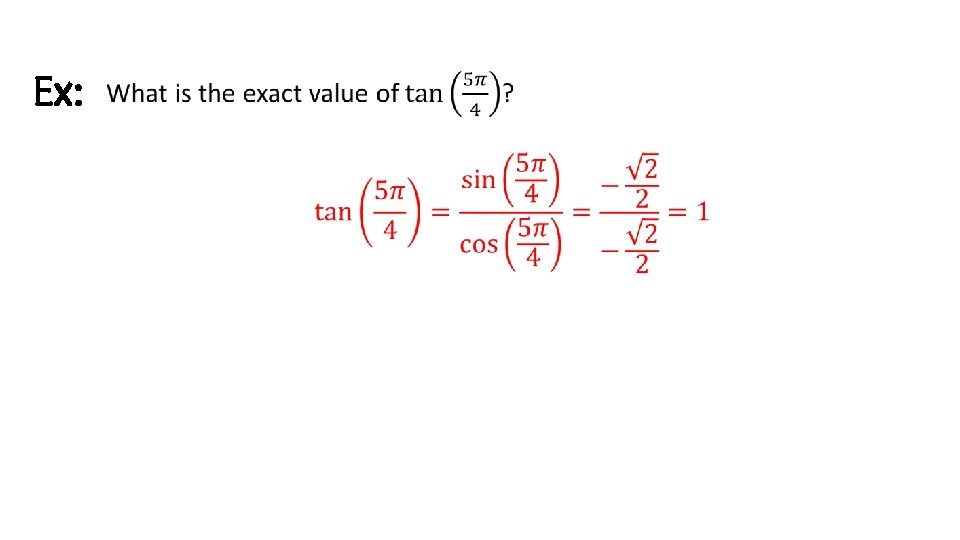Pythagorean Identity •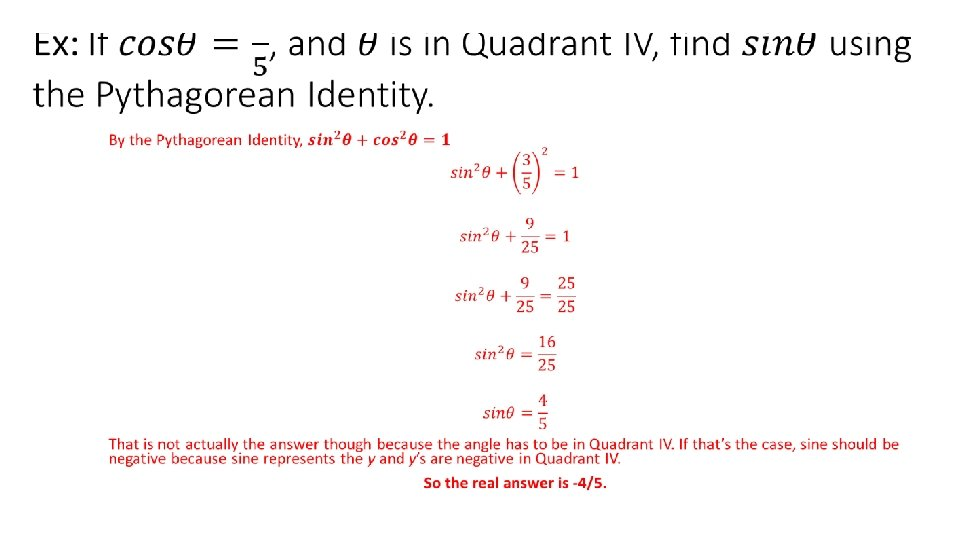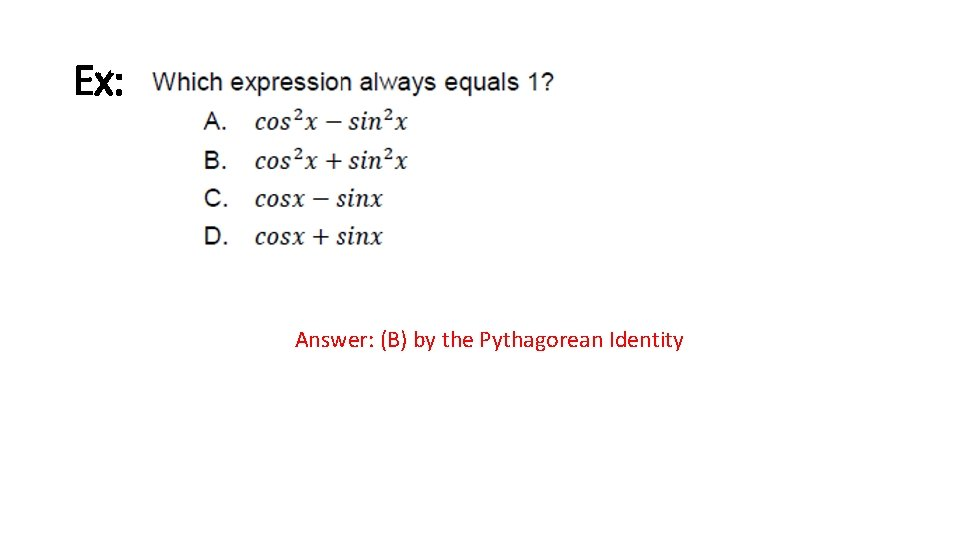Ex: Answer: (B) by the Pythagorean IdentityEx: Answer: (C) by the Pythagorean Identity and after factoringCycles of Sine and Cosine •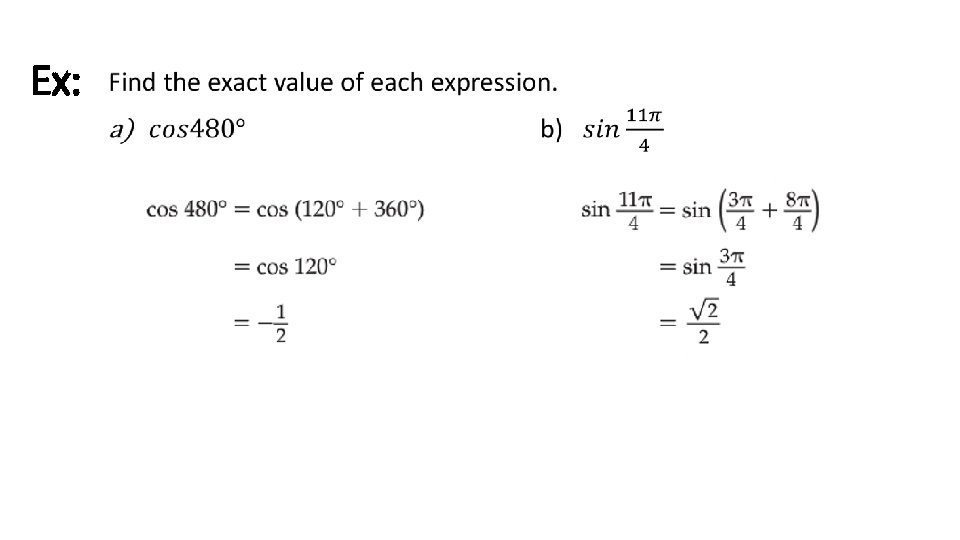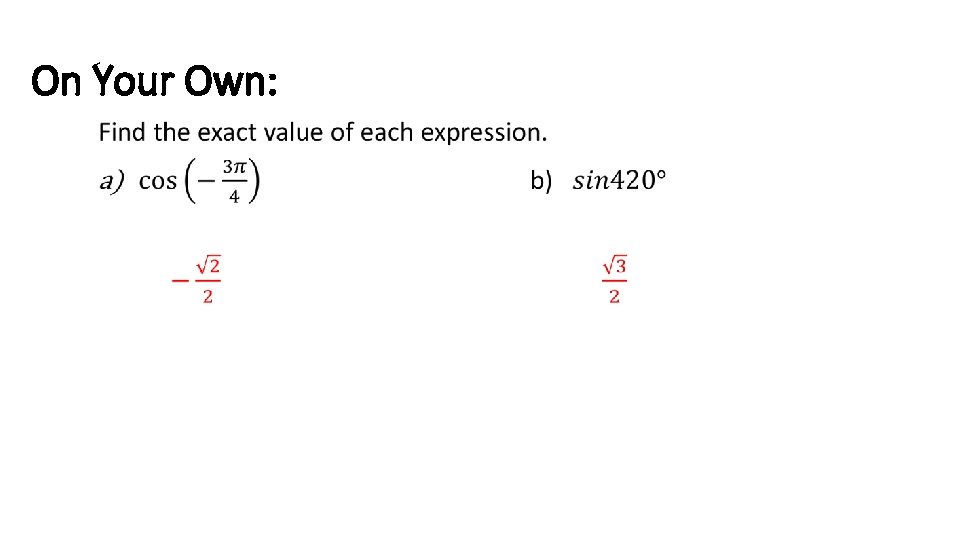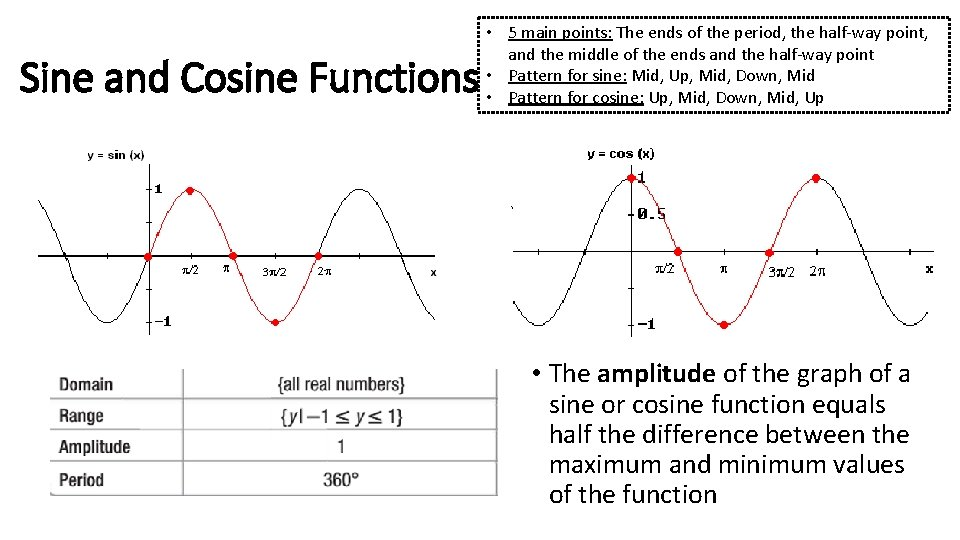Sine and Cosine Functions • 5 main points: The ends of the period, the half-way point, and the middle of the ends and the half-way point • Pattern for sine: Mid, Up, Mid, Down, Mid • Pattern for cosine: Up, Mid, Down, Mid, Up • The amplitude of the graph of a sine or cosine function equals half the difference between the maximum and minimum values of the function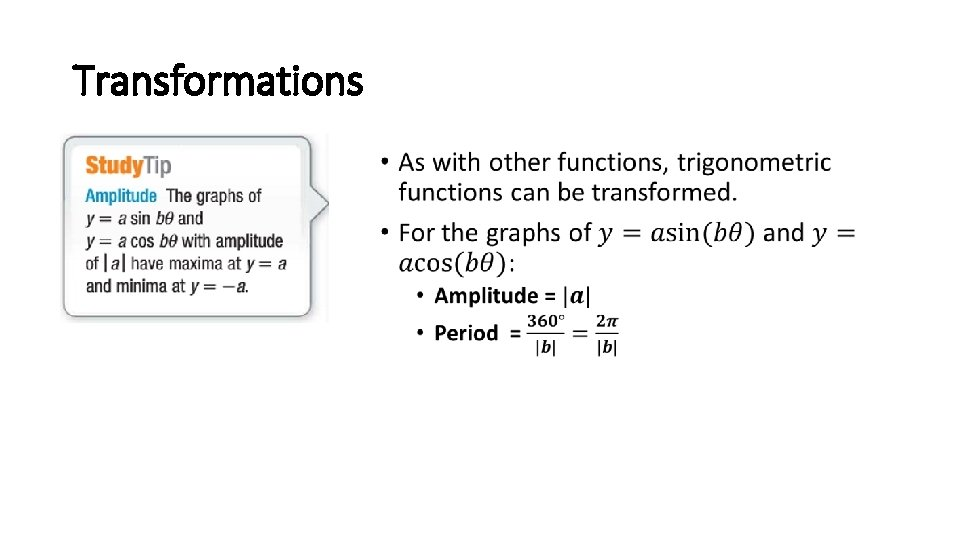Transformations •Ex: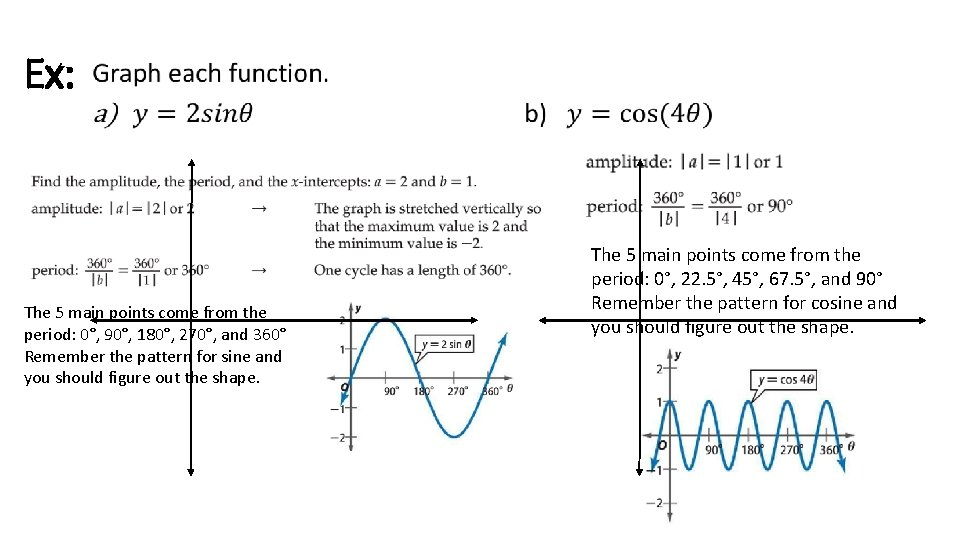Ex: • The 5 main points come from the period: 0°, 90°, 180°, 270°, and 360° Remember the pattern for sine and you should figure out the shape. The 5 main points come from the period: 0°, 22. 5°, 45°, 67. 5°, and 90° Remember the pattern for cosine and you should figure out the shape.